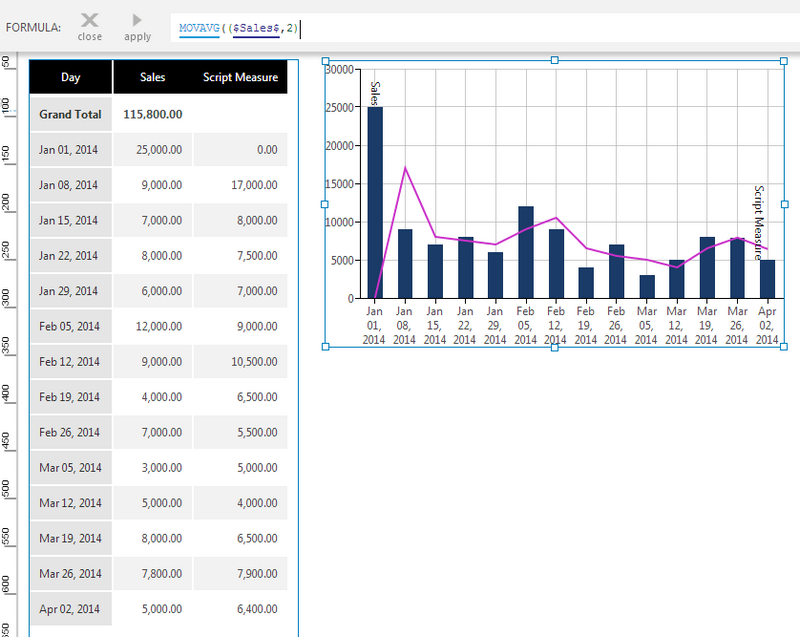# Moving Average

Contents[Hide]

The Moving Average (MA) function computes the average of a set of input values over a specified number of periods. The smaller the number of periods, the faster the moving average responds to changes in the input values.

The mathematical expression being calculated is as follows:

`MAt = (Yt + Yt-1 + Yt-2 + ... + Yt-N+1) / N`

Where N is the number of time periods and Y is the value. You can find further details about the calculation of a moving average from Wolfram MathWorld.Moving Average

## 1. Syntax

```MOVAVG(d0,s0,Alignment)
```

## 2. Input

The Moving Average function requires the following input:

• d0 - Input data values - The set of data values for which the Moving Average is calculated.

## 3. Parameters

The Moving Average function requires the following parameters.

• s0 - The number of periods to use in the calculation. The default value is 10.
• Alignment (Optional) – Hierarchy placeholder to be used as the alignment axis.

## 4. Output

The Moving Average function generates the following output:

• Moving Average - The Moving Average result set.

## 5. See also

Dundas Data Visualization, Inc.
500-250 Ferrand Drive
Toronto, ON, Canada
M3C 3G8

North America: 1.800.463.1492
International: 1.416.467.5100

Dundas Support Hours: 7am-6pm, ET, Mon-Fri